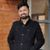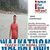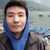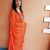# About liquidity ratio, debt to equity ratio, and profitability ratio along with equation & numerical example for each of those

Define ratio analysis- explain liquidity ratio, debt to equity ratio, and profitability ratio. Please give an equation for each (using words to clearly state what to put in the numerator and the denominator of each equation). Provide numerical example for each ratio (each example must have two numbers to compare and state which of the two numbers is more desirable and WHY?) Critique your class member’s postings.

This post was part of TyroCity discussion forumAngel Paudel

From a financial statement, if two or more line items are taken and joined by a mathematical operation, it’s called as the financial ratio. The health or the performance of the company is analyzed with the use of ratio analysis (Gombola & Ketz, 1983). Liquidity, debt and efficiency/profitability are three prominent categories looked into a financial ratio.

Liquidity is the ability of a company to turn their assets into cash. A company wanting to put new investment for the production of their mobile phones for it is doing really well but they don’t have cash in hand, what a company can do now is sell out assets that isn’t used much to invest back on the production. This process of converting assets into cash and the ability of how well, how quick they can do is called as liquidity. This sees for the short-term ability of the company to pay off debt with what the business has (Kregel, 1986).

There are different liquidity ratio. The most popular one of it all is the current ratio with a quick ratio and cash ratio being the other two. The current ratio sees how well the company is able to pay current liabilities with the assets the business have. It is calculated by dividing current assets with current liabilities.

i.e. Current Ratio = Current Assets / Current Liabilities

Table 1:

Company A Company B
Current Assets 2000 2000
Current Liabilities 1800 2500
Current Ratio 2000/1800 = 1.11 2000/2500 = 0.80

From the Table 1, we can observe that the Current ratio of Company A is 1.11 while Company B is 0.8. It’s clearly visible that the ratio is higher for Company A than the other one. The result states that the company A has \$1.11 as current assets for each dollar of their current liabilities while for the company B is \$0.80 as current assets for each dollar of their current liabilities. Company B doesn’t have enough assets to pay off their liabilities while Company A does have more than enough to pay off their current liabilities with their current assets and is thus more desirable.

If a company wants to take a loan to expand and looks out to a bank. The banks now check through the financial record of the company to assess whether or not the company would be able to pay back the debt. The bank would try to ensure what assets are financed by debt and to what level to make an informed decision. This ratio (debt to equity) thus shows what percentage of the company’s assets are financed with the help of debt. It helps in looking into the long-term debt of the company (Auerbach & King, 1983). The calculation of it is done by taking total debt or liabilities divided by total equity or assets the business owns.

i.e. Debt to equity ratio = total liabilities / total assets

The higher the ratio, the more debt the company owns.

Let’s take a simple example to understand it further:

Table 2:

Company A Company B
Total Liabilities 4500 5000
Total Equity 7500 4000
Debt/Equity Ratio 0.60 1.25

From the Table 2, we can observe that the debt to equity ratio of Company A is 0.6 while Company B is 1.25. Company A clearly has a lower debt to equity ratio. A company having a lesser debt ratio means that the company has lesser debt financing and doesn’t have much risk or interest expenses compared one with a higher ratio. This is why Company A is more desirable as it has more equity comparatively.

Profitability ratio helps in measuring the performance of the company by measuring the profits that a company makes. This looks into the ability of a company to determine how well they’re using the assets to generate sales. There are five different types of profitability ratio as in gross profit margin ratio, net profit margin, return on investment ratio, return on stakeholder’s equity ratio, and return on equity (Nissim & Penman, 2001). We look into the gross profit margin ratio alone for the following discussion post. Gross profit margin ratio is used to measure the company’s gross profit over its net sales and is calculated by getting a difference of gross profit and cost of goods sold and then dividing it by the net sales.

i.e. Gross Profit Margin Ratio = (Gross Profit or Sales - Cost of Goods Sold) / Net Sales

Table 3:

Company A Company B
Total Revenue 100000 100000
Cost of Sales 60000 70000
Gross Profit 100000 - 60000 = 40000 100000 - 70000 = 30000
Gross Profit Margin 40000 / 100000 = 40% 30000 / 100000 = 30%

From the Table 3, we can observe that the gross profit margin for Company A is 40% while it’s 30% for Company B. The higher the gross profit margin is, the more profit a company can make off from an individual sale, totaling to higher overall revenue. This is why the 40% is better than 30% of gross profit margin and Company A’s margin is more desirable/better.

References

Auerbach, A., & King, M. (1983). Taxation, Portfolio Choice, and Debt-Equity Ratios: A General Equilibrium Model. The Quarterly Journal Of Economics , 98 (4), 587.

Gombola, M., & Ketz, J. (1983). Financial Ratio Patterns in Retail and Manufacturing Organizations. Financial Management , 12 (2), 45.

Kregel, J. (1986). A Note on Finance, Liquidity, Saving, and Investment. Journal Of Post Keynesian Economics , 9 (1), 91-100.

Nissim, D., & Penman, S. (2001). Review Of Accounting Studies , 6 (1), 109-154.The relation between two numerical figures expressed mathematically is known as ratio. Similarly, analysis of the relationship between various items of financial statements is called financial ratio analysis which helps to summarize the large quantity of financial data and to make quantities judgments about the firm’s financial performance. Financial ratio reflects the quantitative relationship which helps the manager to take qualitative judgment. It can be expressed in terms of percentage, times and proportion or quotient (Barnas, 2007). For example, return is expressed in percentage; turnover is explained in times and so on.

Ratio is very useful for the purpose of taking decision from simple analysis to empirical research in predicting the performance of the company to show the direction of success or failure. The ratio analysis involves comparison of various ratios of the firm with industry average at the same time or with the previous year’s ratio of the firm. It is necessary for useful interpretation of the financial statements. A single ratio in itself does not give any base for managerial decision. To determine the financial condition and performance of the firm, its ratio may be compared with average ratio to the industry of which the firm is involved (Clausen, 2009). Another way of ratio analysis is comparison of the current ratios with the past ratios or with the future or the projected ratios.

1. Liquidity Ratios
Liquidity ratios measure the firm’s ability to satisfy its short terms commitments out of current or liquid asserts. These ratios focuses on current assets and liabilities and are used to ascertain the short term solvency position of the firm. The two primary tests of liquidity are current ratio and quick ratio.

- Current Ratio
A current ratio CR is the quantitative relation between current asserts (CA) and current liabilities (CL). Here, current asserts are those, which can normally be converted into cash within a year. They include cash, inventories, receivables, bank balance, prepaid expenses, and marketable securities and so on.

Current ratio is calculated as follow:

CR = Current asserts/ Current liabilities

Example, For Annapurna Textile Inc. the current asset is Rs. 750000 and the current liabilities is Rs. 700000 hence, the current ratio of the year 2018 is

CR = Rs. 750000/Rs. 700000
= 1.07

The current ratio, 1.07 times indicate that the current asserts of Annapurna Textiles is 1.07 times of its current liabilities.

- Quick Ratio
Quick ratio (QR), also termed as acid - test ratio or liquid ratio, is another measure of short - term solvency of a firm. Quick ratio is defined as the quantitative relationship between quick the

QR = Quick assert/ Current liabilities

Example, For Annapurna Textile Inc. quick assert is Rs. 555000 (CA - Inventory = Rs. 750000 - Rs. 200000) hence the quick ratio for the year 2018 is:

QR = Rs. 550000/ Rs. 700000
= 0.79 times

The quick ratio of 0.79 times that Annapurna Textile’s quick asserts are 0.79 times of current liabilities.

2. Debt - equity ratio
Dept- equity ratio (DE) is the most widely used widely used leverage ratio to equivalent the long term solvency of a firm. The ratio expresses the relationship between debt capital and equity capital, and reflects the relative claim of them on the asserts of the firm. It is simple calculated by dividing total dept by total equity:

DE ratio = Total dept/ Total equity

In the above relationship total dept includes all items of depts, viz., long - term dept and current liabilities. The shareholders’ equity include common equity, preferred stock, and any balance of undistributed profits net of fictitious asserts.

Example, For Annapurna Textile Inc., dept - equity ratio for the year 2018 is

DE ratio = Rs. 1000000/ Rs. 1100000
= 0.9091 times or 90.91 %

Dept - Equity ratio is used as a tools for analyzing financial risk both by creditors as well as by the firm. A higher the dept - equity ratio indicates greater contribution at a firm’s financing by dept holders than those of equity holders.

Profitability Ratios
Profitability is the end result of a number of corporate policies and decisions. It measures how effectively the firm is being operated and managed. Besides owners and managers, creditors are also interested to know the financial soundness of the firm. Owner are eaged to know their returns whereas managers are interested in their operating efficiency. So they calculate profitability ratios because expectations of both owners and managers are evaluated in terms of profit earned by the firm. Following are the major ratios used to measure the profitability of the firm:

Net Profit Margin

Gross Profit Margin

Ø Net profit margin

Net profit margin is the ratio between net income and sales of a firm. It shows the firm’s ability to generate net income per rupee of sales and is calculate as

Net Profit Margin = Net Income/ Sales

Example, For Annapurna Textile Inc., the net profit margin is:

Net Profit margin = Rs. 203000/ Rs. 1500000
= 0.1353 = 13.53%

It means Annapurna Textiles has been able to earn 13.35 percent net profit on its sale. Higher net profit margin is preferred by the owners, the management as well as the creditors.

Ø Gross Profit margin
It is the ratio between gross profit and sales of a firm and is calculated as:

Gross Profit margin = Gross profit/ Sales

Example, the gross profit margin of Annapurna Textile is:

Gross profit margin = Rs 600000/ 1500000
=0.4 or 40%

It means Annapurna Textile earned, after meeting cost of goods sold, 40 percent on its sales. Higher gross profit margin is preferred as it allows greater cushion to absorb other expenses.

References
Barnas, P. (2007). The Analysis and Use of Financial Ratio. Journal of Business Finance & Accounting.

Clausen, J. (2009). Accounting 101 - Financial Statement Analysis in Accounting: Liquidity Ratio Analysis Balance Sheet Assets and Liabilities. Journal of a financial statementFinancial statement of a company is limited to the numbers presented but cannot be analyzed for their trend, its capacity to pay short and long term debt, compare growth between various years or compare performance between two similar companies. Every simple analysis helps interpret operation of a company. To understand these financial ratio can be the turning point in excelling in your business. (Anonymous, 2006)The ratio analysis helps investors to interpret what the company is doing and how they are performing. There are various ratios for the analysis but here we will look into three particular ratios with examples.

Table 1:

Liquidity Ratio: This ratio calculates the liquidity position or the capacity of a company to meet its immediate cash needs. There are various ratios under this. Let us discuss on some of them.

Current Ratio: This ratio is used to calculate whether a company has current assets available to meet the demand of the current liability. It is calculated by dividing current assets by current liability.

Let us take two companies,

Table 1:

Current Assets (CA) in million (NPR) Current Liabilities (CL) in million (NPR) Current Ratio: CA/CL
Company A 30 20 1.5 times
Company B 45 25 1.8 times

From the above calculation we can see that company A has 1.5 times the asset to pay for 1 liability. Similarly company B has 1.8 times the asset to pay for 1 liability. From this ratio we should choose company B as it has more capacity to meet its current liability. This also depends on the type of company. Having high CA is also not good as this fund is nonproductive and contributes less revenue.

Quick Ratio: Quick ratio also calculates the same thing as current ratio but in this ratio we deduct the inventory. This show a company’s liquidity status more than the current asset as inventory takes time to be sold.

Table 2: All figures are in millions of NPR

Assets Company A Company B
Cash 500 450
A/R 700 600
Inventory 250 300
Other CA 250 400
Total CA 1700 1750
Net Fixed Asset 2000 1500
Total Assets 3700 3250
Liabilities
A/P 250 300
N/P 50 70
Other CL 850 1000
Total CL 1150 1370
Long term debt 800 500
Total CL 1950 1870
Quick Ratio 1.2 times 0.82 times
Cash Ratio 0.43 times 0.32 times

We calculate quick ratio as (Current Assets - Inventory)/Current Liabilities

The bench mark for quick ratio is 1:1. In the above example the quick ratio of company A & B are 1.2 times and 0.82 times respectively. Company A has the capacity to meet its liabilities but company B does not so A is better performer.

Cash Ratio: This ratio calculates even higher liquidity position of a company. It is calculated as Cash/Current Liabilities. Referring to table 2, we can see that the cash ratio of Company A & B are 0.43 times and 0.32 times. Since this ratio does not have a bench mark we interpret it as Company A having 0.43 times the capacity to pay it immediate current liability while Company B only has 0.32 times the capacity to pay its current liability.

Net Working Capital to Total Assets

This calculates the net working capital to total asset ratio meaning that it shows how much percent of total asset is the working capital which is there to meet the current liability demand. It is calculated as

Total CA- Total CL / Total Assets

Referring to Table 2, we get,

For Company A

1700-1150/3700 = 14.86%

For Company B

1750-1370/3250 = 11.69%

This shows that total asset of Company A has more percentage of working capital in it than Company B.

Debt to Equity Ratio

Debt to Equity Ratio analyses how much a company has used its investment from debt or equity. What we can analyze from this is that if in case of solvency, the company will prioritize payment to owner or debtor. This is calculated as Total Debt/Total Equity. In the case of Company A and B, referring to Table 2, we can compute that A & B has a debt to equity ratio of 1.14 times and 1.06 times. This shows that A has 1.14 time more debt than equity and B has 1.06 times more debt than equity.

Profitability Ratio

Profitability ratio calculates three different ratio which have been explained below.

Profit Margin: This ratio calculates the net income of the owner. The gross income will have payments to be made but profit margin ratio helps to ascertain the actual income of the owner and is calculated by dividing net income by sales.

Let’s assume, the total revenue is Rs. 6000 million and Net income is Rs. 700 million. Then using the formula we get,

700/6000 = 11.66%. This means that out of Rs. 100 the actual net income of the owner is Rs. 11.66 only.

Return on assets (ROA): This is calculated as net income divided by total assets. It helps to calculate how much net income has been generated by the assets. Taking the above rates of Net income of Rs. 700 million and Total assets of Rs. 3700 million from table 2 we get

700/3700 = 18.91 %.

This means that out of the total net income, total asset has contributed to 18.91%.

Return on Equity: Similar to ROA this calculates the contribution to net income by total equity. Taking Net income as Rs. 700 million and Total Equity as Rs. 1950 from table 2 we get

700/1950 = 35.89 for company A and 700/1870 = 37.43% for Company B.

This two examples show that Company A has less contribution to the net income from the use of equity as compared to company B. "The problem with ROE is that it says nothing about what risks a company has taken to generate its ROE. Because ROE looks only at return while ignoring risk, it can be an inaccurate indicator of financial performance. (Lesakova, 2007)”

These are methods that can be used to compare and contrast between two different companies’ capacity and performance but we must be aware of its limitation and depends on the type of company as well.

Reference

Anonymous. (2006). The Importance of Financial Ratio and Benchmark Analysis. Supply House Times; Troy , 68-69. Retrieved from proxy.lirn.net/MuseProxyID=mp03/Mu...

Lesakova, L. (2007). Uses and Limitations of Profitability Ratio Analysis in Managerial Practice . Banská Bystrica: Matej Bel University .Ratio analysis is a widely used tool for financial analysis. In ratio analysis, we express the relationship between two individual figures or group of figures connected with each other in logical manner in mathematical term. One figure or group of figure is expressed as numerator while the other is expressed as denominator. Ratio analysis helps to identify the strengths and weaknesses of a business concern by comparing the obtained result with the industry average (Moyer, McGuigan, Rao, & Kretlow, 2012). Ratio analysis helps in assessment of liquidity, solvency, profitability and efficiency of a form by calculating different ratios.

Liquidity Ratio: Liquidity or short term solvency is the ability of business to pay its short term liabilities. It is used to analyze the firm’s ability to meet current financial obligations. It shows the relation of cash and other current assets to its current liabilities. The commonly used liquidity ratios are:

Current Ratio: This ratio is calculated by dividing current assets by current liabilities. It indicates the extent to which current liabilities are covered by assets expected to be converted into cash in near future. Current assets include cash, marketable securities, account receivables and inventory. Similarly current liabilities include account payable, short-term notes payable, accrued expenses and long-term debt maturing in current year (Ghimire, 2011).

Current Assets = Current Assets/Current Liabilities

Quick Ratio: Quick ratio shows the ability to meet the current liabilities with most liquid assets. It is also known as acid test ratio. In quick ratio, the inventory is deducted from current assets with the assumption that we cannot make money by selling the inventory whenever we need cash.

Quick Ratio = (Current Assets - Inventory)/Current Liabilities

Cash Ratio : Cash Ratio shows the ability of a firm to meet the current obligations with the available cash balance if the other current assets cannot be converted to cash immediately. It shows how capable we are if we need to pay the current liabilities immediately.

Cash Ratio= (Cash + Marketable Securities) /Current Liabilities

Example

Particulars Company A Company B
Cash 2,000 3,000
Inventory 7,200 6,000
Marketable Securities 1,500 2,000
Account Receivable 4,000 5,000
Total Current Assets 14,700 16,000
Short term Loan 3,500 4,000
Account Payable 4,500 3,500
Accrued Wages 1,500 1,400
Other Accruals 2,000 2,500
Total Current Liabilities 11,500 11,400
Current Ratio 1.28 1.40
Quick Ratio 0.65 0.88
Cash Ratio 0.30 0.44

From the above calculation, we can see that both the companies have enough current assets to meet their current liabilities. Company A and B respectively have assets of Rs.1.28 and Rs.1.40 to pay the current liabilities of Re. 1. Looking at the result of quick ratio, Company B is in better condition than Company A while the result of cash ratio puts Company B in better position. However, the company ratio is used as the bench mark for evaluating the performance. If the calculated ratio is equal to or more than the industry average, the company is considered to be doing well else it needs to revise its business processes.
Debt to Equity Ratio

Debt to equity ratio indicates the proportion of debt fund in relation to equity. This ratio is referred in capital structure decisions. Equity includes common stock, retained earnings and additional paid in capital.it helps to measure the long term solvency of the firm.

Debt to Equity Ratio = Total Debt/Equity

The higher the ratio, riskier would be the capitalization and vice versa.

Example

Company A Company B
Total Liabilities 450,000 450,000
Total Equity 700,000 400,000
Debt/Equity Ratio 0.64 1.13

The debt to equity ratio shows that Company B has more debt financing that increase the interest expenses and risk associated with financing. Thus Company A is better than Company B in this parameter.

Profitability Ratio

The profitability ratio is calculated to measure the operating efficiency of the company. The creditors and owners are interested in the profitability ratio besides the management. This ratio reflects the final results of business operations. Different profitability ratios are:

Gross Profit Margin Ratio: It reflects the average spread of cost over goods sold and sales revenue. A high gross ratio is a sign of good management that indicates the efficiency t o produce goods and services at low cost.

Gross Profit Margin = Gross Profit/Sales

Net Profit Margin: It measures the firm’s ability to turn each rupees sales into profit. A high net profit margin signifies efficient management.

Net Profit Margin = Net Profit/Sales

Return on Assets (ROA): It measures the overall effectiveness or organization in generating profit from available total assets. Higher ROA indicates better performance.

ROA = Net Income/Total Assets

Return on Equity (ROE): It measures the rate of return on stock holder’s investment. A high ROE implies better performance.

ROE = Net Income/Total Equity

Example

Company A Company B
Total Revenue (Sales) 550,000 550,000
Less: Cost of Sales 280,000 350,000
Gross Profit 270,000 200,000
Less: Other Expenses 190,000 125,000
Net Profit 80,000 75,000
Total Assets 1,000,000 950,000
Equity 450,000 550,000
Gross Profit Margin 49.09% 36.36%
Net Profit Margin 14.55% 13.64%
ROA 8% 7.89%
ROE 17.77% 13.64%

From the above calculation, we can see that out of the sales of Re 1, Company A generates 49.09% gross profit and 14.55% net profit. Similarly, Company B makes 36.36% gross profit and 13.64% net profit out of the total sales. Company A is preferable for investment based on ROA and ROE.

References

Ghimire, S. R. (2011). Fundamentals of Financial Management. Kathmandu: K.P Pustak Bhandar.

Moyer, R. C., McGuigan, J. R., Rao, R., & Kretlow, W. J. (2012). Contemporary Financial Management (12th ed.). Oklahoma: Cengage Learning.